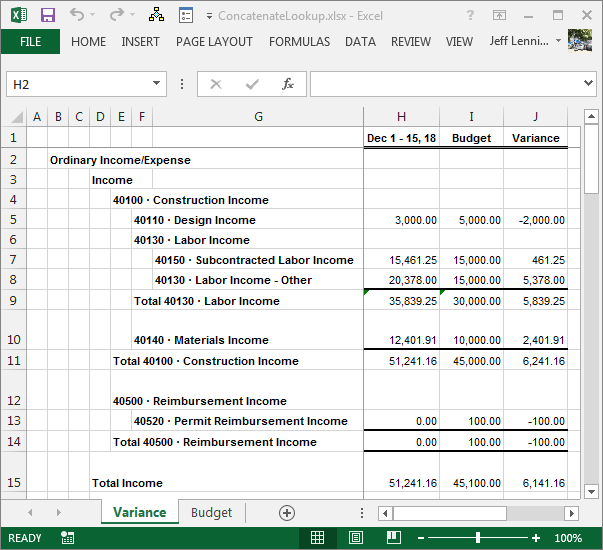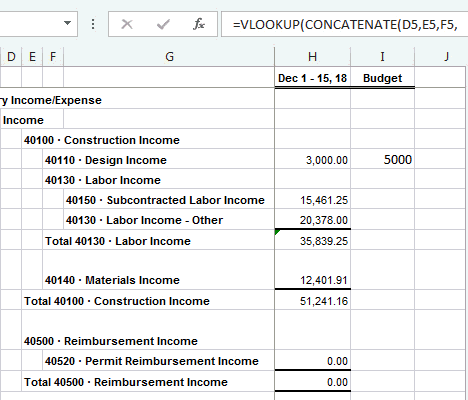# Pull Budget Values into an Income StatementIn this post, we explore a way to pull budget values into an income statement exported from QuickBooks, and demonstrate how to handle the fact that the extract uses new columns to indent.

## Overview

When the budget and actual data reside in the same application, creating a variance report is easy. However, when the actual data is stored in an accounting system, and the budget data is stored in Excel or another application, creating variance reports is a bit more manual. We’ll explore how to use the CONCATENATE and VLOOKUP functions to accomplish the task of pulling budget data into the income statement. For illustration purposes, I used QuickBooks to generate the income statement.

## Variance Report

Let’s take a quick peek at the export that came out of QuickBooks:The goal is to pull the budget data into column I in this income statement.

Let’s take a quick look at the budget data, which is stored in a table named tbl_bud:So, our objective is to write a formula in column I on the income statement that retrieves the values from the budget table.

If we closely examine the income statement format for a moment, we’ll notice that the lookup values are not all stored in the same column. For example, the label for account 40110 is stored in column F, but the label for account 40150 is stored in column G. This makes it difficult to write a formula that we can fill down that will work for all accounts.

To demonstrate the problem, let’s say we wrote the following formula in cell I5:

`=VLOOKUP(F5, tbl_bud, 2, 0)`

Since the report label is in cell F5, the formula works great. However, when we copy this formula down to cell I7 to retrieve the next account, the formula does not work. Excel automatically updates the formula to this:

`=VLOOKUP(F7, tbl_bud, 2, 0)`

Since the account label is not in F7, it is in G7, the formula breaks when we copy it down, as shown below:Fortunately, this situation is relatively easy to handle with the CONCATENATE function.

The CONCATENATE function joins text strings. The function arguments are combined, and the function returns the resulting string. We can simply modify the first argument of the VLOOKUP function by having Excel first combine the label cells.

If all labels were stored in columns F and G for example, we could modify our formula as follows:

`=VLOOKUP(CONCATENATE(F5,G5), tbl_bud, 2, 0)`

This would tell Excel to combine the values in F5 and G5, and then look up the combined string in the budget table.

If we inspect our income statement from top to bottom, we’ll notice that all of the labels we need to lookup are stored in D, E, F, and G. So, we’ll use the following formula:

`=VLOOKUP(CONCATENATE(D5,E5,F5,G5), tbl_bud, 2, 0)`

When we copy this formula down, it works for all lines, as shown below:To finish the variance report, we copy the formula down throughout the income statement, create a variance column, fill the subtotal formulas from the actual column into the budget column, and copy the formatting from the actual column into the budget and variance columns, as illustrated below:Feel free to download the sample file used to create the screenshots above.

As with anything in Excel, there are many ways to accomplish a goal. If you have an approach you use or prefer, please share it by posting a comment below!

• Sample file: ConcatenateLookup
• Probably a better method to use for recurring-use workbooks is to create a third worksheet in the workbook that stores a variance report that uses formulas to retrieve values from both the actual and the budget sheets. Since it can be difficult to use lookup functions or conditional summing functions to retrieve data when the export uses new columns to indent, you may want to explore changing the export type to a flat format, which can sometimes be created with a CSV export.
• Sometimes, exports from accounting systems may include extra spaces. If the account labels have leading or trailing spaces, wrap a TRIM function around the lookup value to remove them.
• If you try to write formulas in the exported QuickBooks worksheet, and the formula is displayed rather than the formula results, you’ll probably need to first format the column to general or number and then write the formula. If you’ve already written the formula, you may need to change the formatting, and then refresh the formula by hitting F2 and then Enter.
• We used VLOOKUP to accomplish the basic retrieval, although, there are plenty of other options you may prefer including INDEX/MATCH or SUMIFS.

Get a quick email notice when a new Excel article is available

• This field is for validation purposes and should be left unchanged.